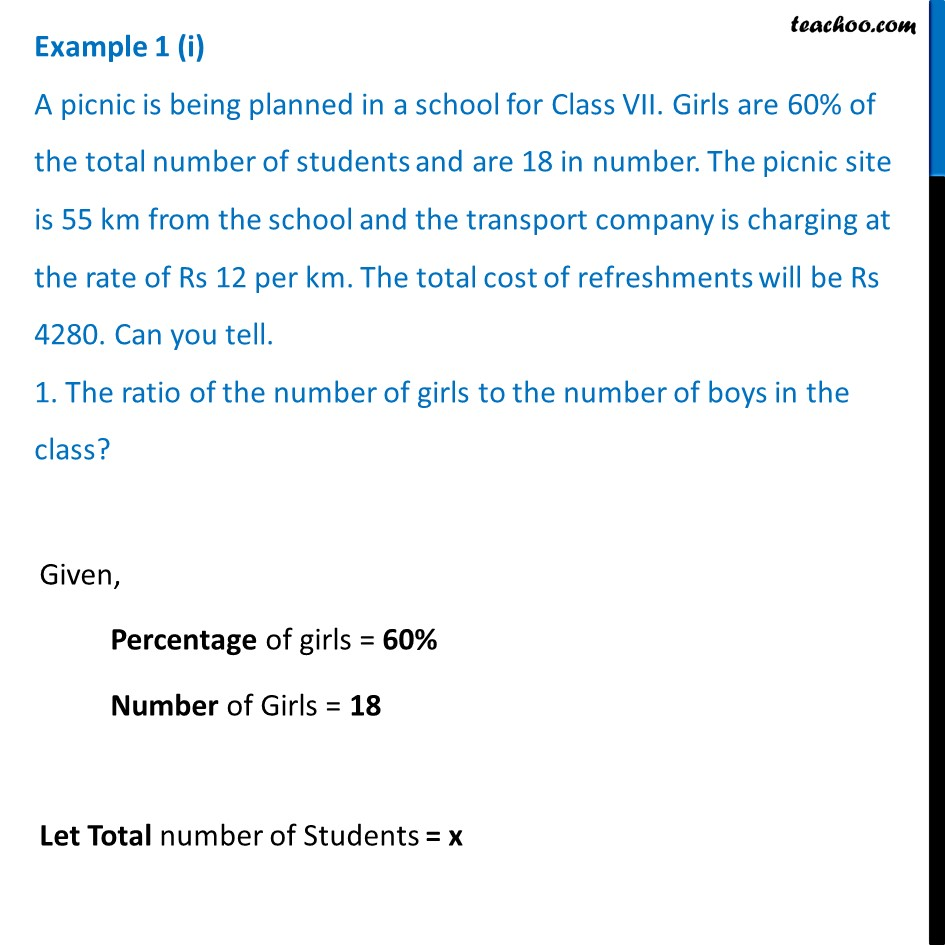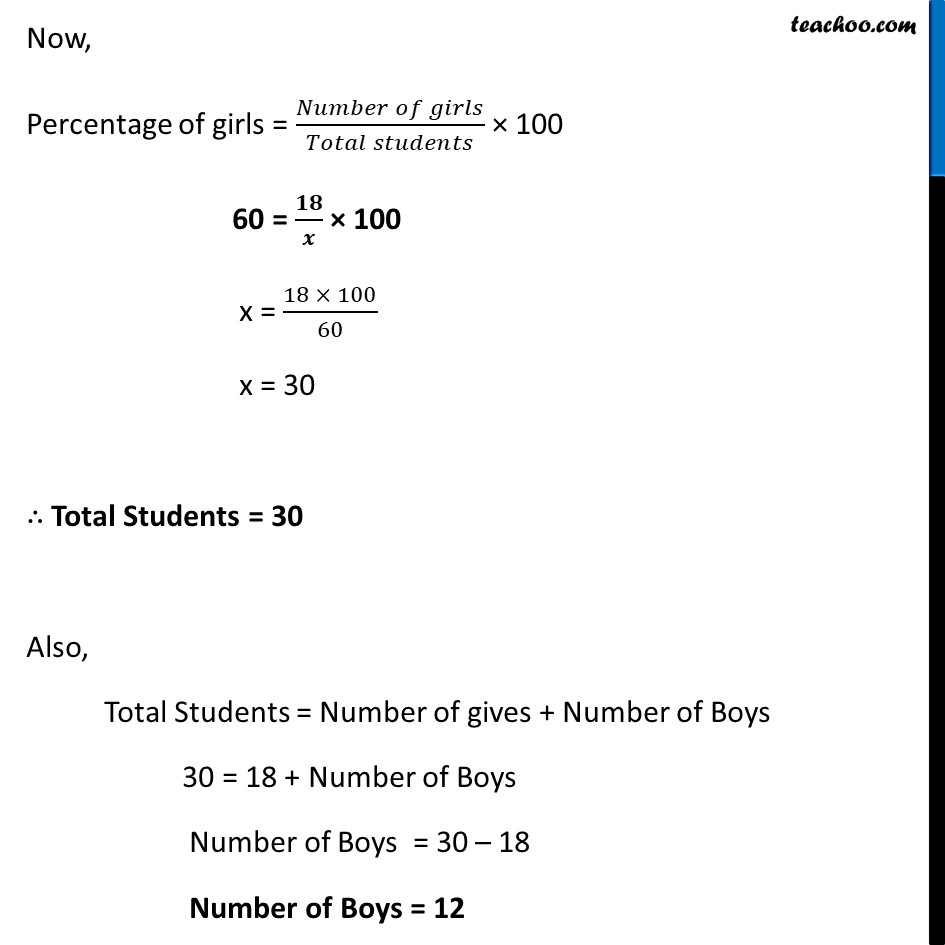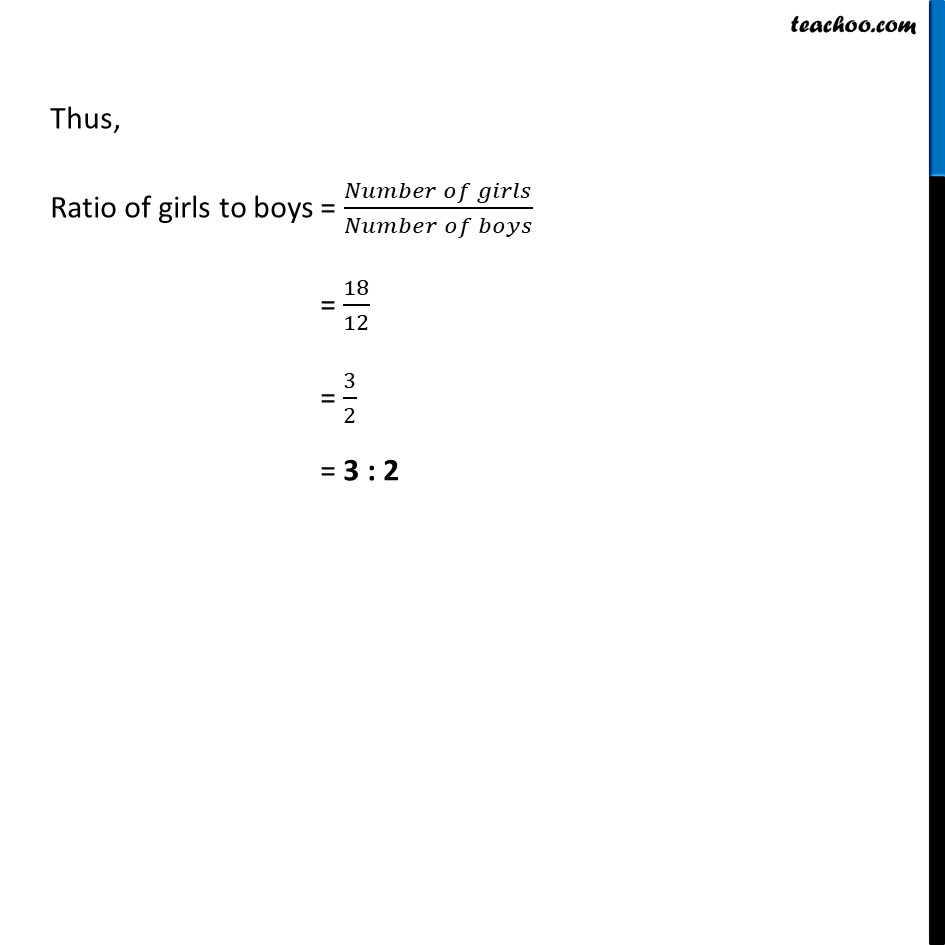Examples

Chapter 7 Class 8 Comparing Quantities
Serial order wiseLearn in your speed, with individual attention - Teachoo Maths 1-on-1 Class

### Transcript

Example 1 (i) A picnic is being planned in a school for Class VII. Girls are 60% of the total number of students and are 18 in number. The picnic site is 55 km from the school and the transport company is charging at the rate of Rs 12 per km. The total cost of refreshments will be Rs 4280. Can you tell. 1. The ratio of the number of girls to the number of boys in the class?Given, Percentage of girls = 60% Number of Girls = 18 Let Total number of Students = x Now, Percentage of girls = (𝑁𝑢𝑚𝑏𝑒𝑟 𝑜𝑓 𝑔𝑖𝑟𝑙𝑠)/(𝑇𝑜𝑡𝑎𝑙 𝑠𝑡𝑢𝑑𝑒𝑛𝑡𝑠) × 100 60 = 𝟏𝟖/𝒙 × 100 x = (18 × 100)/60 x = 30 ∴ Total Students = 30 Also, Total Students = Number of gives + Number of Boys 30 = 18 + Number of Boys Number of Boys = 30 – 18 Number of Boys = 12 Thus, Ratio of girls to boys = (𝑁𝑢𝑚𝑏𝑒𝑟 𝑜𝑓 𝑔𝑖𝑟𝑙𝑠)/(𝑁𝑢𝑚𝑏𝑒𝑟 𝑜𝑓 𝑏𝑜𝑦𝑠) = 18/12 = 3/2 = 3 : 2